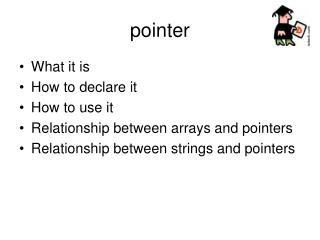DownloadDownload Presentationpointer

# pointer

Télécharger la présentation## pointer

- - - - - - - - - - - - - - - - - - - - - - - - - - - E N D - - - - - - - - - - - - - - - - - - - - - - - - - - -
##### Presentation Transcript

1. pointer • What it is • How to declare it • How to use it • Relationship between arrays and pointers • Relationship between strings and pointers

2. pointer A pointer is a variable that contains a memory address as its value count countPtr 10

3. pointer count countPtr 1000 10 memory address1000 count 10 countPtr 1000

4. How to declare a pointer int * countPtr; countPtr is a variable of type “pointer to int”

5. example int count = 10; int *countPtr = 1000; memory address 1000 count 10 countPtr 1000

6. How to initialize a pointer to point to an address int count = 10; int * countPtr = &count; & is an operator that returns the address of a variable. memory address 1000 count 10 countPtr 1000

7. Restrictions for using & operator • May be used for variables int count; int *countPtr = &count; • May not be used with constants (&10)

8. How to initialize a pointer to point to nothing int * aPtr = NULL; OR int * aPtr; aPtr = NULL;

9. Indirect and direct references Direct reference – changing count memory location using count variable Indirect reference – changing count memory location using countPtr variable countPtr count 10

10. Indirect and direct references int count; int * countPtr = &count; count = 12; // direct reference *countPtr = 12 // indirect reference * is the indirection operator. Means change the memory location currently pointed to by countPtr. countPtr count 12 10

11. Exercises • Declare an integer variable called x. Declare a pointer to x called xPtr and initialize it so that it points to the variable x. Use xPtr to indirectly change the value of x to 20; • Declare a second integer variable called y. Declare a pointer to y called yPtr and initialize it so that it points to the variable y. Calculate the sum of x + y using xPtr and yPtr.

12. pointer arithmetic int *aPtr; aPtr++; // is valid but what does it do? integer (4 bytes) aPtr aPtr is incremented by the size of whatever it is pointing to. In this example 4 bytes. So the value in aPtr is increased by 4 by the aPtr ++ statement

13. pointer arithmetic char *aPtr; aPtr++; char (1 byte) aPtr aPtr is incremented by the size of whatever it is pointing to. In this example 1 byte. So the value in aPtr is increased by 1 by the aPtr ++ statement

15. using pointer arithmetic char letterPtr = &letters; letterPtr ‘a’ letters letters letters letters letters ‘b’ ‘c’ letterPtr ++; letterPtr ‘d’ ‘e’

16. pointers and arrays • array name is a pointer. This is why: • can pass array name to a function without the brackets • arrays are passed to functions as reference parameters by default

17. arrays as function parameters Pass the name of the array without the brackets void PrintResults(int results[ ]); // function prototype void PrintResults(int results[ ]) // function definition { } calling sequence: const int maxRange = 10; int frequency[maxRange ] = {0}; PrintResults (frequency);

18. pointers and arrays int b[ ] = {1,2,3,4,5}; b 1 b b b b b 2 3 4 5

19. pointers and arrays b int *bPtr; int b[ ] = {1,2,3,4,5}; bPtr = &b; or bPtr = b; bPtr 1 b b b b b 2 3 4 5

20. pointers and strings char digits [ ] = {“0123456789”}; digits           ‘0’ ‘1’ ‘2’ ‘3’ ‘4’ ‘5’ ‘6’ ‘7’ ‘8’ ‘9’ NULL

21. pointers and strings char digits [ ] = {“0123456789”}; digits           ‘0’ char *digitsPtr = digits; ‘1’ ‘2’ digitsPtr ‘3’ ‘4’ ‘5’ ‘6’ ‘7’ ‘8’ ‘9’ NULL

22. simplify Instead of char digits [ ] = {“0123456789”}; char *digitsPtr = digits; do char *digitsPtr = “0123456789”;

23. simplify char *digitsPtr = “0123456789”; cout << digitsPtr << endl; // displays all the numbers in the string

24. Simplify Array of strings const int Size = 5; const int WordSize = 10; char article [Size] [WordSize] = {"the", "a", "one", "some", "any"}; char noun [Size] [WordSize] = {"boy", "girl", "dog", "town", "car"}; char verb [Size] [WordSize] = {"drove", "jumped", "ran", "walked", "skipped"}; char preposition [Size] [WordSize] = {"to", "from", "over", "under", "on"};

25. Array of strings const int Size = 5; char *article [Size] = {"the", "a", "one", "some", "any"}; char *noun [Size] = {"boy", "girl", "dog", "town", "car"}; char *verb [Size] = {"drove", "jumped", "ran", "walked", "skipped"}; char *preposition [Size] = {"to", "from", "over", "under", "on"};

26. The End !!!!